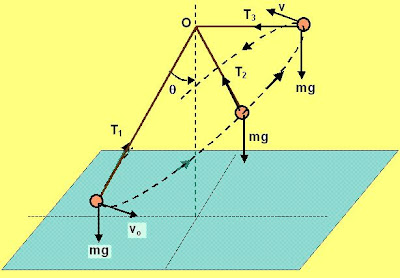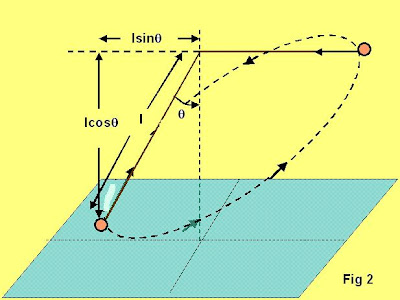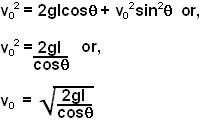## Sunday, June 29, 2008

### Irodov Problem 1.192The balls motion is shown along the dotted path - its a motion in all 3 dimensions and the ball is shown at three positions during its motion - initial (lowest), some intermediate position (middle) and the highest position (when the deflection is 90 degrees). The tension in the thread will be different at all the points of the balls motion in this path. The only constant force acting will be force of gravity mg acting downwards.

As the ball raises up, it will loose its kinetic energy (velocity) and gain potential energy. Let its velocity at the highest point be v. Since this is the highest point, there vertical component of v will be zero (in other words the ball is not moving up any more) .The direction of v will be tangential and along the horizontal plane as shown in the figure. At the highest point the ball has gained a height of(as shown in figure 2) and hence a potential energy of. From conservation of energy we have,Now how to find v?

Consider the torques acting on particle as seen from point O. There are two forces acting on the ball at any time, the tension in the string and the force of gravity. Since tension vector passes through O, there will be no torque generated on the ball due to tension in the string at any point of time. The only torque generated will be due to the force of gravity.Since the force of gravity is always acting downwards, the torque vector L
due to gravity will always be restricted to the horizontal plane as shown in figure 3. In other words at no point during the balls motion, there is any component of torque L acting in the vertical direction as seen from point O. This means that the vertical component of the angular momentum of the ball w.r.t O will always be conserved.

The initial angular momentum of the ball w.r.t point O, is mvol. The vertical component of angular momentum then is. When the ball reaches its maximum height and the string is horizontal the angular momentum will be mvl and this will directed vertically upwards. Since the vertical component of the angular momentum is always conserved we have ,From (1) and (2) we have,